# Comparison Operators in python

So, basically, comparison operators are used to comparing two values that are numbers.

If we level up to be geeky, comparison operators can also be used to compare other data types.

Now, let’s start with equality checks and I hope you like spider-man movies.

## `==` Equal comparison operator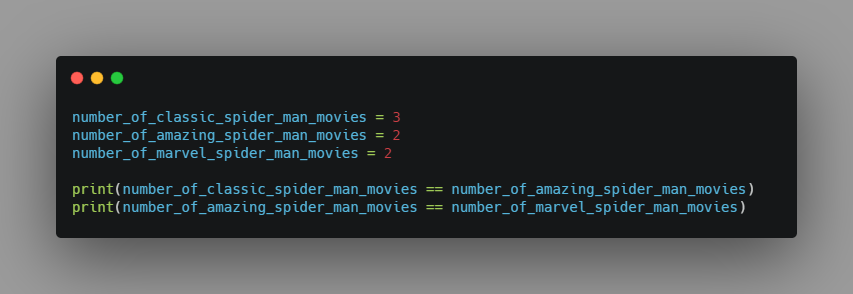Output:

``````False
True
``````

## `!=` Not Equal comparison operator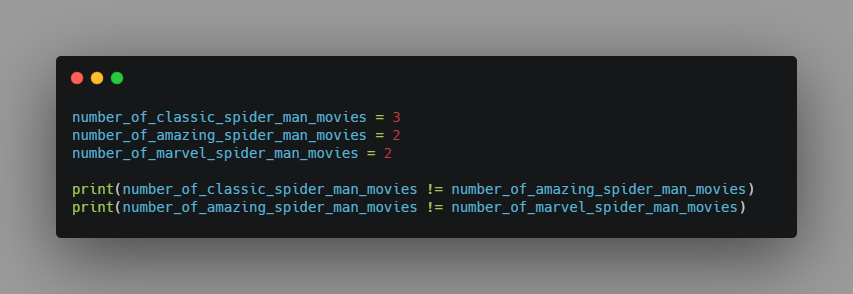Output:

``````True
False
``````

Alright, I’m sure that you are aware of how to use other operators to compare two number values, right? OK, now’s the time to level up to be geeky.

For the rest of the operators let us compare the letters from the Alphabet. Wait, what?! You heard me right!

Let me explain it at the end of this post.

## `>` Greater than comparison operatorOutput:

``````False
True
False
``````

## `<` Less than comparison operator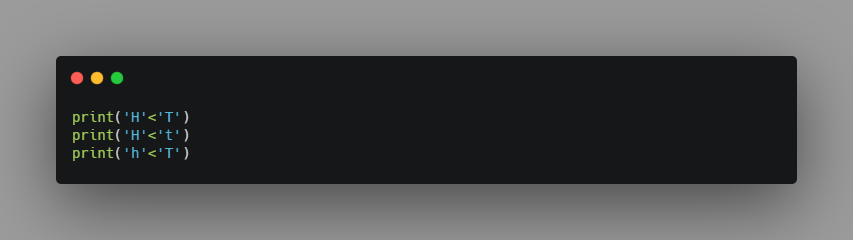Output:

``````True
True
False
``````

## `>=` Greater than or equal to comparison operator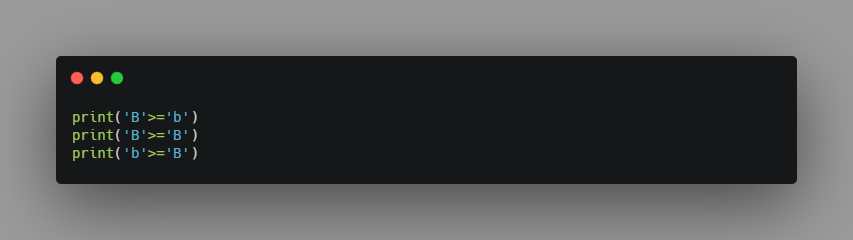Output:

``````False
True
True
``````

## `<=` Less than or equal to comparison operator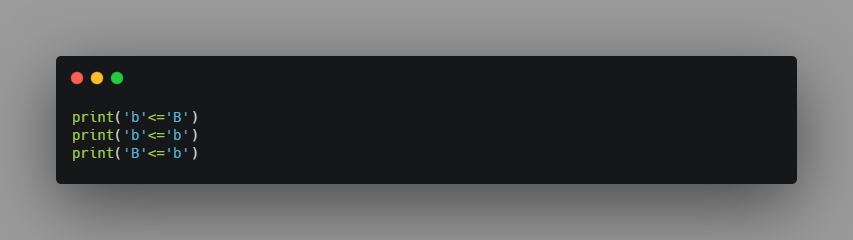Output:

``````False
True
True
``````

Here’s the answer for the above craziness.

When we compare two letters (or characters), it gets converted into ASCII code. You can check the link where the table contains ‘DEC’ (Decimal values) for the characters from Alphabet.

Now that the characters are converted into ASCII code, which is nothing but numbers and we are back to square one. That is, we can compare the values as numbers and return True or false.

### Code along and have fun ;)## Aswin Barath

My name is Aswin Barath. I’m a technical blogger and budding software engineer